# Real numbersPage 1

#### WATCH ALL SLIDES

Slide 1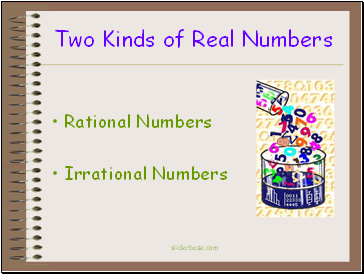Two Kinds of Real Numbers

Rational Numbers

Irrational Numbers

Slide 2## Real numbers

(as opposed to fake numbers?)

Slide 3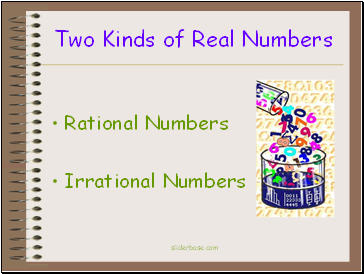Two Kinds of Real Numbers

Rational Numbers

Irrational Numbers

Slide 4## Rational Numbers

A rational number is a real number that can be written as a ratio of two integers.

A rational number written in decimal form is terminating or repeating.

Slide 5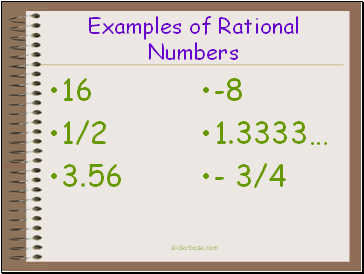Examples of Rational Numbers

16

1/2

3.56

-8

1.3333…

- 3/4

Slide 6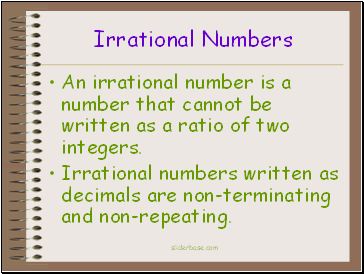## Irrational Numbers

An irrational number is a number that cannot be written as a ratio of two integers.

Irrational numbers written as decimals are non-terminating and non-repeating.

Slide 7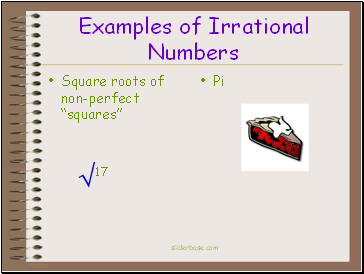Examples of Irrational Numbers

Square roots of non-perfect “squares”

Pi

17

Slide 8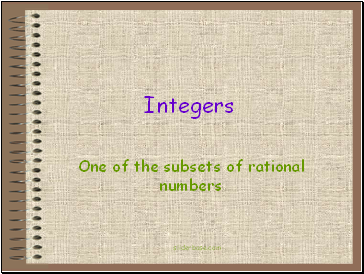## Integers

One of the subsets of rational numbers

Slide 9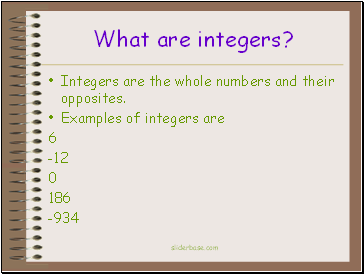What are integers?

Integers are the whole numbers and their opposites.

Examples of integers are

6

-12

0

186

-934

Slide 10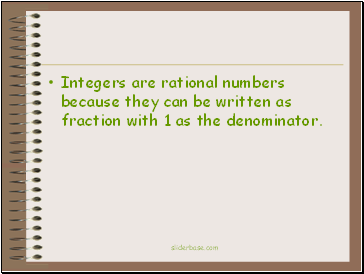Integers are rational numbers because they can be written as fraction with 1 as the denominator.